Home » 30 Questions to test a data scientist on K-Nearest Neighbors (kNN) Algorithm

# 30 Questions to test a data scientist on K-Nearest Neighbors (kNN) Algorithm

## Introduction

If you were to ask me 2 most intuitive algorithms in machine learning – it would be k-Nearest Neighbours (kNN) and tree based algorithms. Both of them are simple to understand, easy to explain and perfect to demonstrate to people. Interestingly, we had skill tests for both these algorithms last month.

If you are new to machine learning, make sure you test yourself on understanding of both of these algorithms. They are simplistic, but immensely powerful and used extensively in industry.  This skill test will help you test yourself on k-Nearest Neighbours. It  is specially designed for you to test your knowledge on kNN and its applications.

More than 650 people registered for the test. If you are one of those who missed out on this skill test, here are the questions and solutions. Here is the leaderboard for the participants who took the test.

Here are some resources to get in depth knowledge in the subject.

If you are just getting started with Machine Learning and Data Science, here is a course to assist you in your journey to Master Data Science and Machine Learning. Check out the detailed course structure in the link below:

## Skill test Questions and Answers

1) [True or False] k-NN algorithm does more computation on test time rather than train time.

A) TRUE
B) FALSE

Solution: A

The training phase of the algorithm consists only of storing the feature vectors and class labels of the training samples.

In the testing phase, a test point is classified by assigning the label which are most frequent among the k training samples nearest to that query point – hence higher computation.

2) In the image below, which would be the best value for k assuming that the algorithm you are using is k-Nearest Neighbor.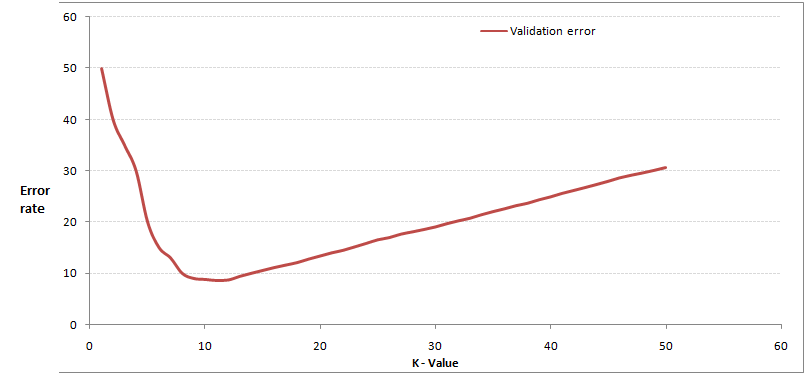A) 3
B) 10
C) 20
D 50

Solution: B

Validation error is the least when the value of k is 10. So it is best to use this value of k

3) Which of the following distance metric can not be used in k-NN?

A) Manhattan
B) Minkowski
C) Tanimoto
D) Jaccard
E) Mahalanobis
F) All can be used

Solution: F

All of these distance metric can be used as a distance metric for k-NN.

4) Which of the following option is true about k-NN algorithm?

A) It can be used for classification
B) It can be used for regression
C) It can be used in both classification and regression

Solution: C

We can also use k-NN for regression problems. In this case the prediction can be based on the mean or the median of the k-most similar instances.

5) Which of the following statement is true about k-NN algorithm?

1. k-NN performs much better if all of the data have the same scale
2. k-NN works well with a small number of input variables (p), but struggles when the number of inputs is very large
3. k-NN makes no assumptions about the functional form of the problem being solved

A) 1 and 2
B) 1 and 3
C) Only 1
D) All of the above

Solution: D

The above mentioned statements are assumptions of kNN algorithm

6) Which of the following machine learning algorithm can be used for imputing missing values of both categorical and continuous variables?

A) K-NN
B) Linear Regression
C) Logistic Regression

Solution: A

k-NN algorithm can be used for imputing missing value of both categorical and continuous variables.

7) Which of the following is true about Manhattan distance?

A) It can be used for continuous variables
B) It can be used for categorical variables
C) It can be used for categorical as well as continuous
D) None of these

Solution: A

Manhattan Distance is designed for calculating the distance between real valued features.

8) Which of the following distance measure do we use in case of categorical variables in k-NN?

1. Hamming Distance
2. Euclidean Distance
3. Manhattan Distance

A) 1
B) 2
C) 3
D) 1 and 2
E) 2 and 3
F) 1,2 and 3

Solution: A

Both Euclidean and Manhattan distances are used in case of continuous variables, whereas hamming distance is used in case of categorical variable.

9) Which of the following will be Euclidean Distance between the two data point A(1,3) and B(2,3)?

A) 1
B) 2
C) 4
D) 8

Solution: A

sqrt( (1-2)^2 + (3-3)^2) = sqrt(1^2 + 0^2) = 1

10) Which of the following will be Manhattan Distance between the two data point A(1,3) and B(2,3)?

A) 1
B) 2
C) 4
D) 8

Solution: A

sqrt( mod((1-2)) + mod((3-3))) = sqrt(1 + 0) = 1

Context: 11-12

Suppose, you have given the following data where x and y are the 2 input variables and Class is the dependent variable.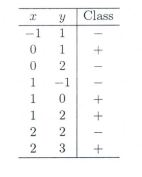Below is a scatter plot which shows the above data in 2D space.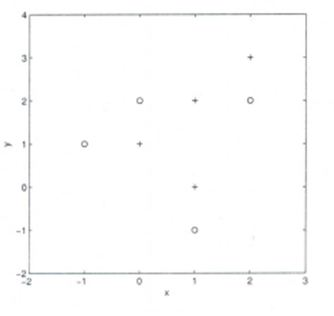11) Suppose, you want to predict the class of new data point x=1 and y=1 using eucludian distance in 3-NN. In which class this data point belong to?

A) + Class

B) – Class

C) Can’t say

D) None of these

Solution: A

All three nearest point are of +class so this point will be classified as +class.

12) In the previous question, you are now want use 7-NN instead of 3-KNN which of the following x=1 and y=1 will belong to?

A) + Class

B) – Class

C) Can’t say

Solution: B

Now this point will be classified as – class because there are 4 – class and 3 +class point are in nearest circle.

Context 13-14:

Suppose you have given the following 2-class data where “+” represent a postive class and “” is represent negative class.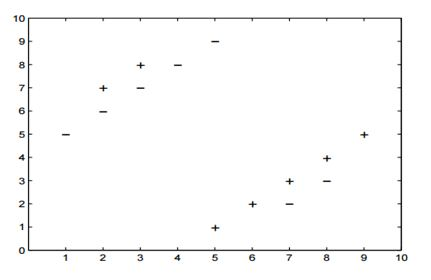13) Which of the following value of k in k-NN would minimize the leave one out cross validation accuracy?

A) 3
B) 5
C) Both have same
D) None of these

Solution: B

5-NN will have least leave one out cross validation error.

14) Which of the following would be the leave on out cross validation accuracy for k=5?

A) 2/14
B) 4/14
C) 6/14
D) 8/14
E) None of the above

Solution: E

In 5-NN we will have  10/14 leave one out cross validation accuracy.

15) Which of the following will be true about k in k-NN in terms of Bias?

A) When you increase the k the bias will be increases
B) When you decrease the k the bias will be increases
C) Can’t say
D) None of these

Solution: A

large K means simple model, simple model always condider as high bias

16) Which of the following will be true about k in k-NN in terms of variance?

A) When you increase the k the variance will increases
B) When you decrease the k the variance will increases
C) Can’t say
D) None of these

Solution: B

Simple model will be consider as less variance model

17) The following two distances(Eucludean Distance and Manhattan Distance) have given to you which generally we used in K-NN algorithm. These distance are between two points A(x1,y1) and B(x2,Y2).

Your task is to tag the both distance by seeing the following two graphs. Which of the following option is true about below graph ?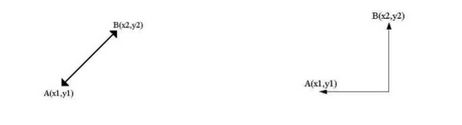A) Left is Manhattan Distance and right is euclidean Distance
B) Left is Euclidean Distance and right is Manhattan Distance
C) Neither left or right are a Manhattan Distance
D) Neither left or right are a Euclidian Distance

Solution: B

Left is the graphical depiction of how euclidean distance works, whereas right one is of Manhattan distance.

18) When you find noise in data which of the following option would you consider in k-NN?

A) I will increase the value of k
B) I will decrease the value of k
C) Noise can not be dependent on value of k
D) None of these

Solution: A

To be more sure of which classifications you make, you can try increasing the value of k.

19) In k-NN it is very likely to overfit due to the curse of dimensionality. Which of the following option would you consider to handle such problem?

1. Dimensionality Reduction
2. Feature selection

A) 1
B) 2
C) 1 and 2
D) None of these

Solution: C

In such case you can use either dimensionality reduction algorithm or the feature selection algorithm

20) Below are two statements given. Which of the following will be true both statements?

1. k-NN is a memory-based approach is that the classifier immediately adapts as we collect new training data.
2. The computational complexity for classifying new samples grows linearly with the number of samples in the training dataset in the worst-case scenario.

A) 1
B) 2
C) 1 and 2
D) None of these

Solution: C

Both are true and self explanatory

21) Suppose you have given the following images(1 left, 2 middle and 3 right), Now your task is to find out the value of k in k-NN in each image where k1 is for 1st, k2 is for 2nd and k3 is for 3rd figure.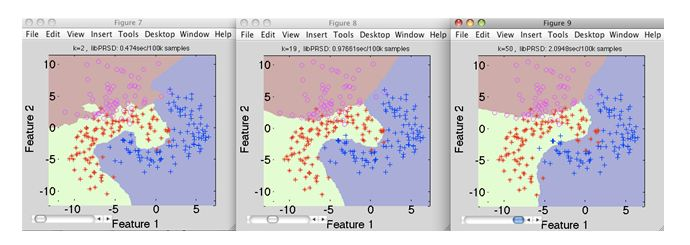A) k1 > k2> k3
B) k1<k2
C) k1 = k2 = k3
D) None of these

Solution: D

Value of k is highest in k3, whereas in k1 it is lowest

22) Which of the following value of k in the following graph would you give least leave one out cross validation accuracy?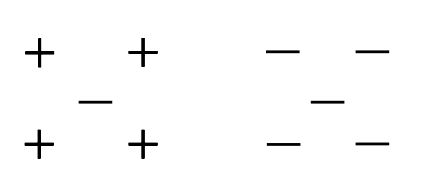A) 1
B) 2
C) 3
D) 5

Solution: B

If you keep the value of k as 2, it gives the lowest cross validation accuracy. You can try this out yourself.

23) A company has build a kNN classifier that gets 100% accuracy on training data. When they deployed this model on client side it has been found that the model is not at all accurate. Which of the following thing might gone wrong?

Note: Model has successfully deployed and no technical issues are found at client side except the model performance

A) It is probably a overfitted model
B) It is probably a underfitted model
C) Can’t say
D) None of these

Solution: A

In an overfitted module, it seems to be performing well on training data, but it is not generalized enough to give the same results on a new data.

24) You have given the following 2 statements, find which of these option is/are true in case of k-NN?

1. In case of very large value of k, we may include points from other classes into the neighborhood.
2. In case of too small value of k the algorithm is very sensitive to noise

A) 1
B) 2
C) 1 and 2
D) None of these

Solution: C

Both the options are true and are self explanatory.

25) Which of the following statements is true for k-NN classifiers?

A) The classification accuracy is better with larger values of k
B) The decision boundary is smoother with smaller values of k
C) The decision boundary is linear
D) k-NN does not require an explicit training step

Solution: D

Option A: This is not always true. You have to ensure that the value of k is not too high or not too low.

Option B: This statement is not true. The decision boundary can be a bit jagged

Option C: Same as option B

Option D: This statement is true

26) True-False: It is possible to construct a 2-NN classifier by using the 1-NN classifier?

A) TRUE
B) FALSE

Solution: A

You can implement a 2-NN classifier by ensembling 1-NN classifiers

27) In k-NN what will happen when you increase/decrease the value of k?

A) The boundary becomes smoother with increasing value of K
B) The boundary becomes smoother with decreasing value of K
C) Smoothness of boundary doesn’t dependent on value of K
D) None of these

Solution: A

The decision boundary would become smoother by increasing the value of K

28) Following are the two statements given for k-NN algorthm, which of the statement(s)

is/are true?

1. We can choose optimal value of k with the help of cross validation
2. Euclidean distance treats each feature as equally important

A) 1
B) 2
C) 1 and 2
D) None of these

Solution: C

Both the statements are true

Context 29-30:

Suppose, you have trained a k-NN model and now you want to get the prediction on test data. Before getting the prediction suppose you want to calculate the time taken by k-NN for predicting the class for test data.
Note: Calculating the distance between 2 observation will take D time.

29) What would be the time taken by 1-NN if there are N(Very large) observations in test data?

A) N*D
B) N*D*2
C) (N*D)/2
D) None of these

Solution: A

The value of N is very large, so option A is correct

30) What would be the relation between the time taken by 1-NN,2-NN,3-NN.

A) 1-NN >2-NN >3-NN
B) 1-NN < 2-NN < 3-NN
C) 1-NN ~ 2-NN ~ 3-NN
D) None of these

Solution: C

The training time for any value of k in kNN algorithm is the same.

## Overall Distribution

Below is the distribution of the scores of the participants: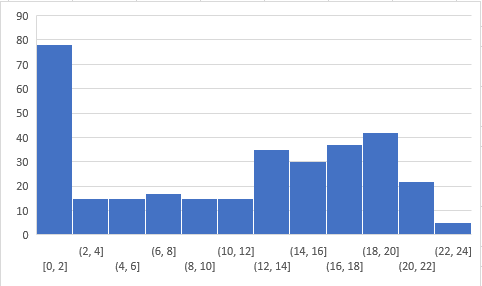You can access the scores here. More than 250 people participated in the skill test and the highest score obtained was 24.

## End Notes

I tried my best to make the solutions as comprehensive as possible but if you have any questions / doubts please drop in your comments below. I would love to hear your feedback about the skill test. For more such skill tests, check out our current hackathons.

### Learn, engage,compete, and get hired!

You can also read this article on our Mobile APP•Rafsanjani Muhammod says:

WOW !!
Nice Sir, Thankyou.

•Sasikanth says:

Answer to Q 12 should be + class. All together there are 8 observation 4 of – and 4 of+. But the nearest ones for 1,1 is +

•Faizan Shaikh says:

Hi Sasikanth,

There was a typo in the questions. I have updated the same, thanks for the feedback

•Anubhav says:

•Aishwarya Singh says:

Hi Anubhav,

Answer to Q 25 is that the decision boundary can be a bit jagged( not smooth when k is small). Whereas the answer to Q25 is that the decision boundary is smooth for large values of k.

•Nenad says:

10 is wrong.

•Aishwarya Singh says: